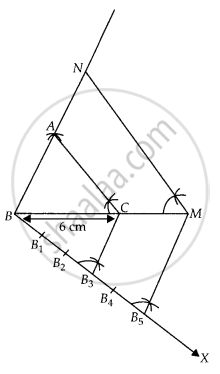# Draw a triangle ABC in which BC = 6 cm, CA = 5 cm and AB = 4 cm. Construct a triangle similar to it and of scale factor 53. - Mathematics

Diagram
Sum

Draw a triangle ABC in which BC = 6 cm, CA = 5 cm and AB = 4 cm. Construct a triangle similar to it and of scale factor 5/3.

#### Solution

Steps of construction:

1. Draw a line segment BC = 6 cm.

2. Taking B and C as centres, draw two arcs of radii 4 cm and 5 cm intersecting each other at A.

3. Join BA and CA. ∆ABC is the required triangle.

4. From B, draw any ray BX downwards making at acute angle ∠CBX

5. Mark five points B1, B2, B3, B4 and B5 on BX, such that BB1 = B1B2 = B2B3 = B3B4 = B4B5.6. Join B3C and from B5 draw B5M ॥ B3C intersecting the extended line segment BC at M.

7. From point M draw MN ॥ CA intersecting the extended line segment BA at N.

Then, ∆NBM is the required triangle

Whose sides is equal to 5/3 of the corresponding sides of the ∆ABC.

Hence, ∆NBM is the required triangle.

Concept: Division of a Line Segment
Is there an error in this question or solution?

#### APPEARS IN

NCERT Mathematics Exemplar Class 10
Chapter 10 Construction
Exercise 10.3 | Q 3 | Page 116
Share